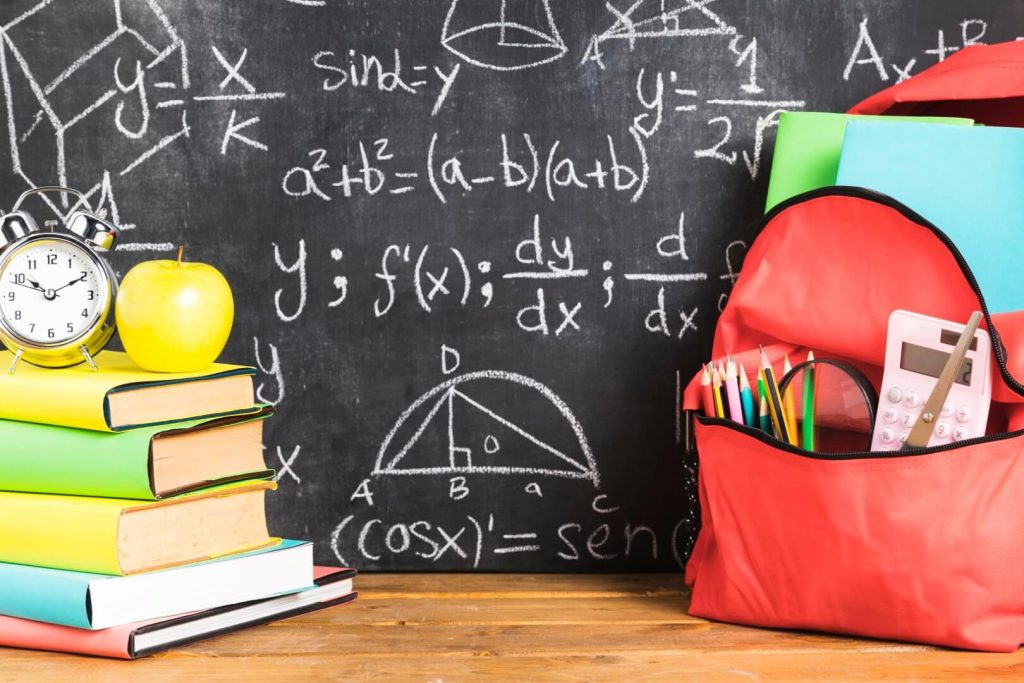# Algebra Basics

In this article, we are going to learn some algebra basics including,

• What are the algebraic symbols?
• Why do we use algebraic symbols?
• How to construct an algebraic expression for a given instance.

At this moment, some of you may have an idea about algebra. Some may not.

Let’s start from the beginning.

## Introduction to Algebra Basics

We use simple letters in the English alphabet as algebraic symbols. We usually use letters like x, y, z, a, b, c, m, n, p, q, etc.

we use these symbols to denote Unknown Constant Values and Variables.

This example shows where we can use an algebraic symbol to represent an unknown constant.

## Examples for Unknown Constant Values

Example 01:

If Kevin buys the same amount of milk every day and if the amount is unknown, we cannot express the amount numerically.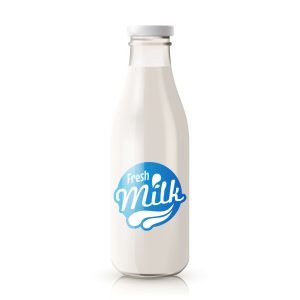At that moment, we have to use an Algebraic Symbol to represent the amount.

You can choose any letter you like. Let’s take the amount of milk that Kevin buys as ‘x

Example 02:

It is an unknown constant.We can use an algebraic symbol to denote the number of words. You can use any letter you like.

I am going to take the number of words in this article as ‘n‘.

### Examples for Variables

Example 03:The distance traveled by car with 1 gallon of diesel.

The distance will differ with road conditions and some other factors.We cannot say the distance accurately with a number.

At this moment, we have a variable. To indicate a variable, you can use an algebraic symbol.

I am going to take the distance as ‘p‘ miles.

## Building Algebraic Expressions

Algebraic expressions are mathematical expressions consisting of algebraic symbols, mathematical operations, and numerical values.

## Algebra Basics Examples

Example 01:

The number of pencils in a pencil box is ‘x’. Then I added 3 more pencils to the pencil box.

Now, what will be the total number of pencils in the pencil box?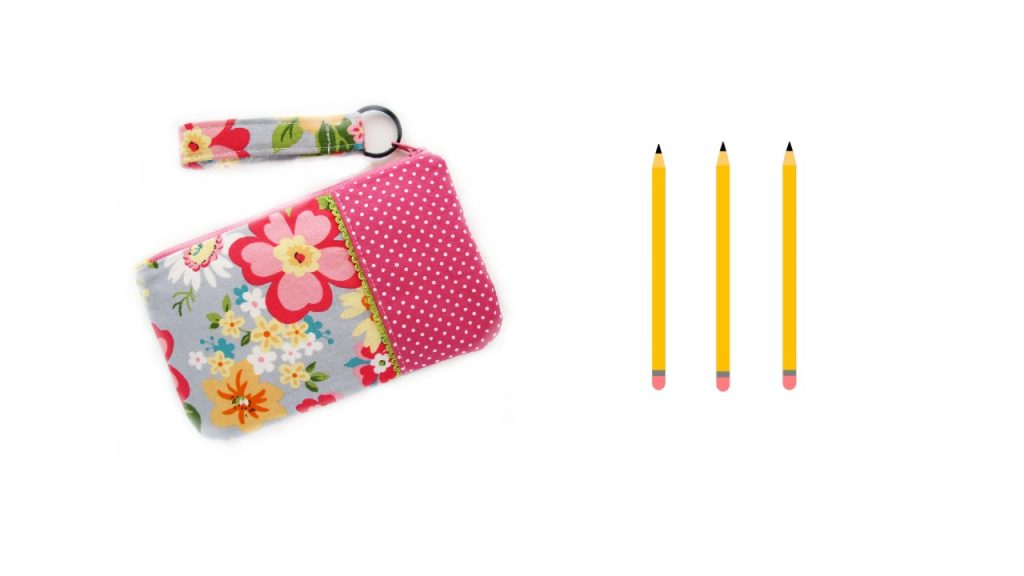Total number of pencils in the box = x + 3

x + 3 is an Algebraic Expression.

Example 02:

I have a candy jar. But I don’t know how much candies in that jar. Let’s express the total number of candies as ‘y’.Then I ate 10 candies from it. Now let’s find the remaining number of candies in the jar.

Remaining number of candies        =    y – 10

Example 03:

There are ‘m’ number of books in one box. There are 9 other boxes contain the same amount of books in each box.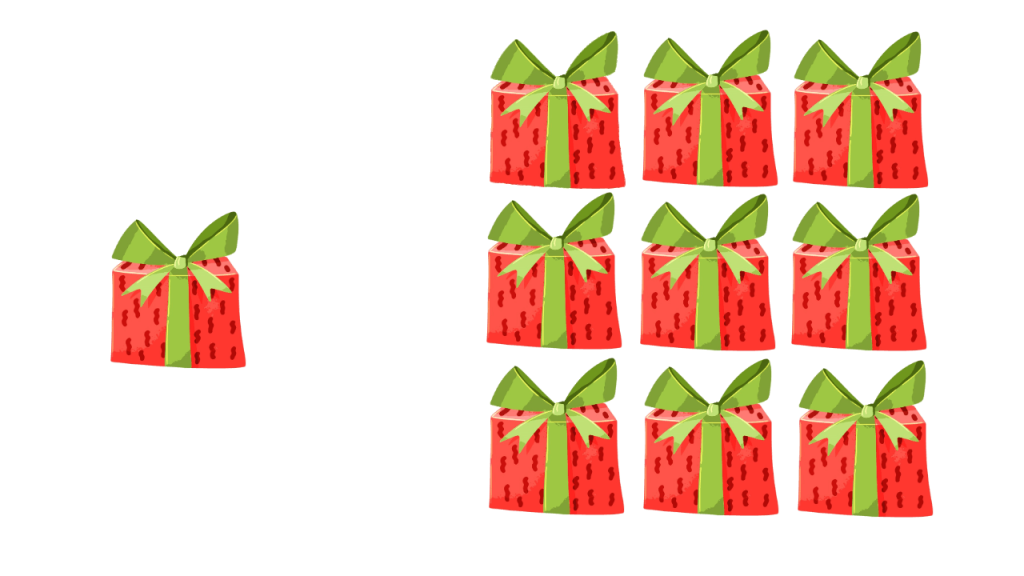How many books are in all 9 boxes?

We can work on this problem in two ways.

m+m+m+m+m+m+m+m+m = 9m

02. Multiply the number of books containing in one box by the number of boxes.

9 x m = 9m

According to the basic algebra rules, we can eliminate the multiplication sign and write the answer as 9m.

Example 04:

I’m ‘t’ years old and my sister is half of my age. Find my sister’s age.To get my answer I have to divide my age by 2.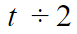According to the basic rules, we can eliminate the division sign and write the sister’s age as,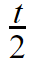### 4 thoughts on “Algebra Basics”

1.why cant we have class work on this stuffs to test our ability

2.so easy

3.Nicely described. Easy to understand. Thank you.